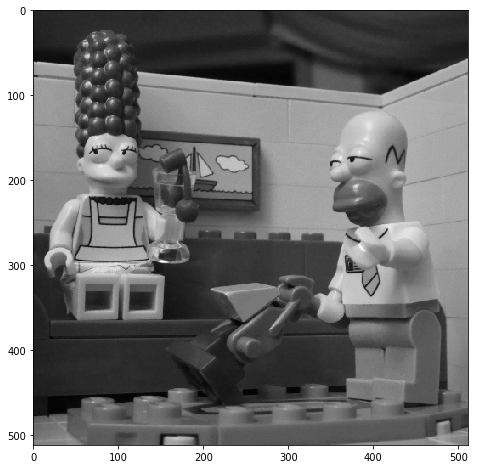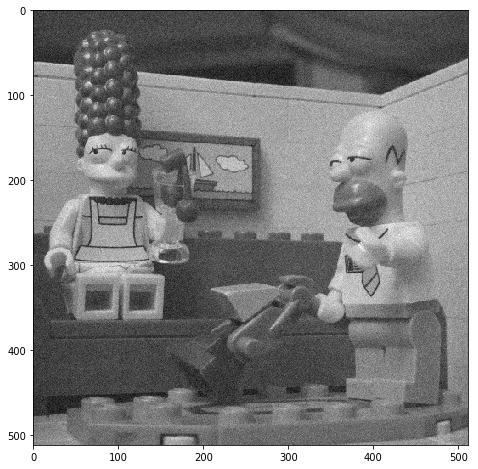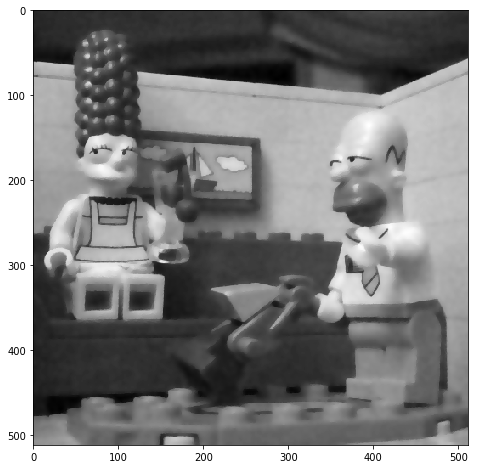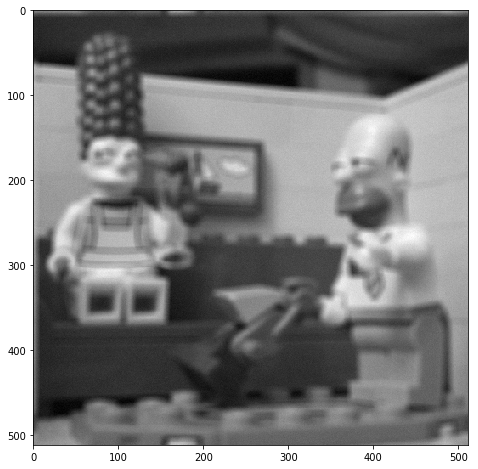In :
# IF YOU WANT TO USE GOOGLE DRIVE FOR THIS PRACTICAL SESSION :

In :
import numpy as np
import matplotlib.pyplot as plt
from TVL2 import * # solutions


# TVL2 - reminder¶

In the following, we propose to restore an image thanks to a variationnal TV+L2 model. The observed image $u_b$ is a degraded version of an (unknown) image $u$ through the model : $$u_b = Au + b$$ with $A$ a known linear operator, $b$ a noise image, realisation of a random image with normal distribution $\mathcal{N}(0,Id).$$The TV-L2 optimization problem we wish to solve is the following$$\mathrm{argmin}_u \;\;\frac 1 2 \|Au-u_b\|_2^2+\lambda \mathrm{TV}(u)\quad (\mathcal{P}).$$To this aim, we first rewrite the problem as a saddle point problem$$\mathrm{arg}\min_u \max_p\underbrace { \frac 1 2 \|Au-u_b\|_2^2+ \; <\lambda \nabla u, p > \;- \iota_{\|.\|_{\infty,i}}(p)}_{H(u,p)}$$and we apply the Chambole-Pock algorithm to solve this optimization problem. The algorithm is essentially a proximal gradient descent applied to the function H(u,p), alternatively in u and p, with an extra gradient step. ALGORITHM • u_0,p_0 initialization • iterate •$$p_{k+1} = \mathrm{prox}_{-\tau H_{u_k}} (p_k) =\pi_{\|.\|_{\infty,2}}(p_k+\tau \lambda \nabla \bar{u}_k)$$•$$ u_{k+1} = \mathrm{prox}_{\sigma H_{p_{k+1}}} (u_k) = (Id+\sigma A A^*)^{-1}\left(u_k +\sigma A^* u_b + \lambda \sigma \mathrm{div}(p_k)\right) $$•$$\bar{u}_{k+1} = u_{k+1} + \theta(u_{k+1} - {u}_k)$$To tis aim, we will need two functions to compute the divergence of a vector field and the gradient of an image. These discretization schemes for these two functions should be chosen such that \mathrm{div}(\mathrm{grad}) is a discretization of the laplacian operator. In : def div(cx,cy): #cy and cy are coordonates of a vector field. #the function compute the discrete divergence of this vector field nr,nc=cx.shape ddx=np.zeros((nr,nc)) ddy=np.zeros((nr,nc)) ddx[:,1:-1]=cx[:,1:-1]-cx[:,0:-2] ddx[:,0]=cx[:,0] ddx[:,-1]=-cx[:,-2] ddy[1:-1,:]=cy[1:-1,:]-cy[0:-2,:] ddy[0,:]=cy[0,:] ddy[-1,:]=-cy[-2,:] d=ddx+ddy return d  In : def grad(im): #compute the gradient of the image 'im' # image size nr,nc=im.shape gx = im[:,1:]-im[:,0:-1] gx = np.block([gx,np.zeros((nr,1))]) gy =im[1:,:]-im[0:-1,:] gy=np.block([[gy],[np.zeros((1,nc))]]) return gx,gy  ## TVL2 for denoising¶ We create the noisy image. In : u = plt.imread('../im/simpson512.png') # images can be found here https://github.com/judelo/notebooks/tree/master/im u = u[:,:,1] plt.figure(figsize = (8,8)) plt.imshow(u, cmap = 'gray')  Out: <matplotlib.image.AxesImage at 0x115ec3d68>In : # denoising sigmanoise = 0.05 nr,nc = u.shape ub = u+sigmanoise*np.random.randn(nr,nc) plt.figure(figsize = (8,8)) plt.imshow(ub, cmap = 'gray')  Out: <matplotlib.image.AxesImage at 0x1164d1a90>Write a function chambolle_pock_prox_TV implementing the Chambolle-Pock algorithm for TVL2 denoising. The function should take as inputs the noisy image ub, the regularization parameter \lambda and a number of iterations. In : # TVL2 denoising ut = chambolle_pock_prox_TV(ub,0.1,100) #display the result plt.figure(figsize = (8,8)) plt.imshow(ut,cmap='gray')  Out: <matplotlib.image.AxesImage at 0x106701940># TVL2 for deblurring¶ In this section, the observed image u_b can be written$$Au+b$$where the operator A represents a (circular) convolution with a known kernel h. For the sake of simplicity we propose to use a uniform kernel for h but you can try other kinds of kernels. The matrix A is never computed explicitely (it would be HUGE !), all the operations involving A or A^* must be written directly in the Fourier domain. For instance: • Au is a convolution with the kernel h, it can be computed as$$\mathcal{F}^{-1} ( \hat{u}. \hat{h} )$$with$\mathcal{F}$the Fourier transform and$\mathcal{F}^{-1}$the inverse Fourier transform. •$A^*u$is a convolution with the conjugate of the kernel$h$... • the inversion$(Id+\sigma AA^*)^{-1} u $in the Fourier domain becomes a division$\frac{\hat{u}}{1+ \sigma |\hat{h}|^2}$In : s=4 #kernel definition (for a 9x9 uniform kernel) h = np.zeros((u.shape,u.shape)) h[0:2*s+1,0:2*s+1] = np.ones((2*s+1, 2*s+1))/(2*s+1)**2  In : convol = lambda a,b: np.real(np.fft.ifft2(np.fft.fft2(a)*np.fft.fft2(b)))  In : # blurred image ub = convol(u,h) + 0.01*np.random.randn(u.shape,u.shape) plt.figure(figsize = (8,8)) plt.imshow(ub, cmap = 'gray')  Out: <matplotlib.image.AxesImage at 0x1062bca58>Write a function chambolle_pock_deblurring_TV implementing Chambolle-Pock for image deblurring. Try to apply this algorithm to the blurred version of$u$, with or without noise added to the blurred image. How should be chosen the regularization parameter$\lambda\$ in each case?

In :
lambd = 1e-3
niter = 200

# TVL2 deblurring
ut = chambolle_pock_deblurring_TV(ub,h,lambd,niter)

#display result
plt.figure(figsize = (8,8))
plt.imshow(ut,cmap='gray')

Out:
<matplotlib.image.AxesImage at 0x1066454e0>